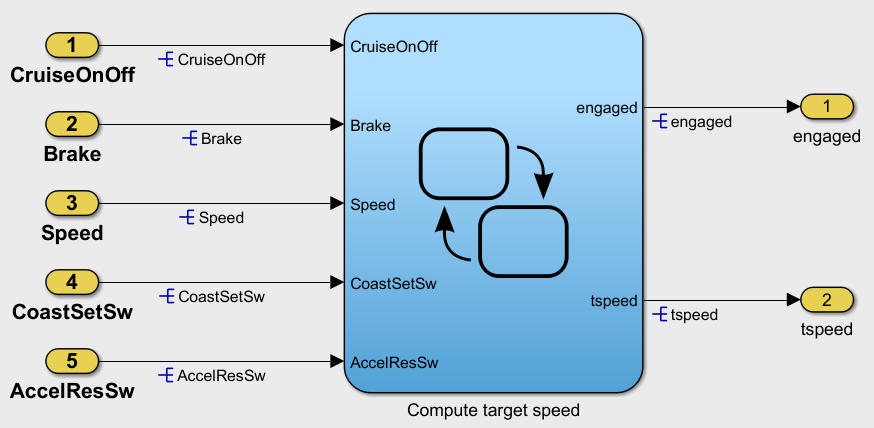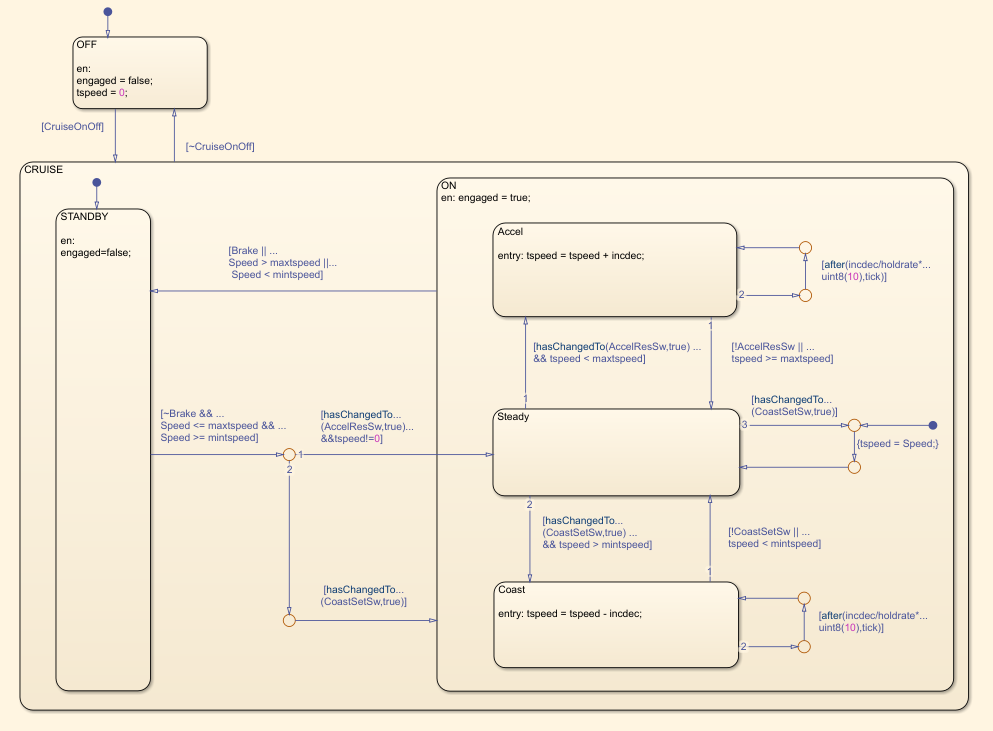## Compare Model Complexity and Code Complexity Metrics

Analyze the complexity of your system by using the cyclomatic complexity metrics. The metrics indicate the structural complexity of a system by measuring the number of linearly independent paths in the system. By limiting the cyclomatic complexity of your system, you can make it more readable, maintainable, and portable. You can measure the cyclomatic complexity for both your model and the code generated from your model. Note that differences between the code and the model may result in different levels of cyclomatic complexity. To measure the cyclomatic complexity of a model, use the Metrics Dashboard and the Cyclomatic complexity metric.

### Metric Threshold Values

#### Code Complexity Threshold

When you develop an algorithm by hand-writing code, you assess the readability of the code by measuring the cyclomatic complexity of the code. Code that has higher cyclomatic complexity can be more difficult to understand and maintain. To standardize code maintainability, your organization may select a threshold value that limits the cyclomatic complexity of your code. For example, if you write code that conforms to the HIS Code Complexity Metrics (Polyspace Bug Finder), you check that the cyclomatic complexity of the code is at or below the threshold of 10.

#### Model Complexity Threshold

When you use the model-based design workflow to model an algorithm and generate code, you can assess the readability of the system by using the cyclomatic complexity metric of the model instead of measuring the cyclomatic complexity of the generated code. The graphical modeling of Simulink® allows you to manage complex algorithms better than traditional hand code does. To account for this, the default cyclomatic complexity metric threshold for the model is 30, which is higher than the standard code complexity threshold of 10. To change the model metric threshold value, see Customize Metrics Dashboard Layout and Functionality.

### Comparing Code and Model Complexity Metric Results

The cyclomatic complexity of a model can be higher or lower than the cyclomatic complexity of the generated code. This variation depends on your model and on your code generation customizations. Some of the patterns that generate different complexity measurements include:

• Code generation optimizations that eliminate extra logic that the model contains. These optimizations can reduce the complexity of the code.

• Error checks in the generated code that the model metric analysis does not consider. These error checks can increase the complexity of the code.

• Additional logic in the generated code for a specific target. This logic can increase the complexity of the code.

For example, consider the model `simulinkCruiseErrorAndStandardsExample`. To open the model:

1. Open the project.

```path = fullfile(matlabroot,'toolbox','shared','examples',... 'verification','src','cruise'); run(fullfile(path,'slVerificationCruiseStart'))```

2. From the project, open the model folder and open `simulinkCruiseErrorAndStandardsExample`.The model contains the chart Compute target speed. To generate and analyze code for the chart by using Polyspace®, see Analyze Code and Test Software-in-the-Loop.The reports that Polyspace generates for the code include code metrics such as cyclomatic complexity. The generated step function for the chart has a cyclomatic complexity of 20.

To measure cyclomatic complexity of the model, use the Metrics Dashboard:

1. Open the Metrics Dashboard. In the Apps gallery, click Metrics Dashboard.

2. Click .

3. To view detailed cyclomatic complexity results, click the Model Complexity widget.

The chart in the model has a cyclomatic complexity of 30. For this chart, the code generator optimizes the code by consolidating logic, so the generated code has a lower cyclomatic complexity than the chart in the model. In other cases, a model may have lower cyclomatic complexity than its generated code. When you maintain the model for code generation, use the cyclomatic complexity of the model to measure your system's complexity.